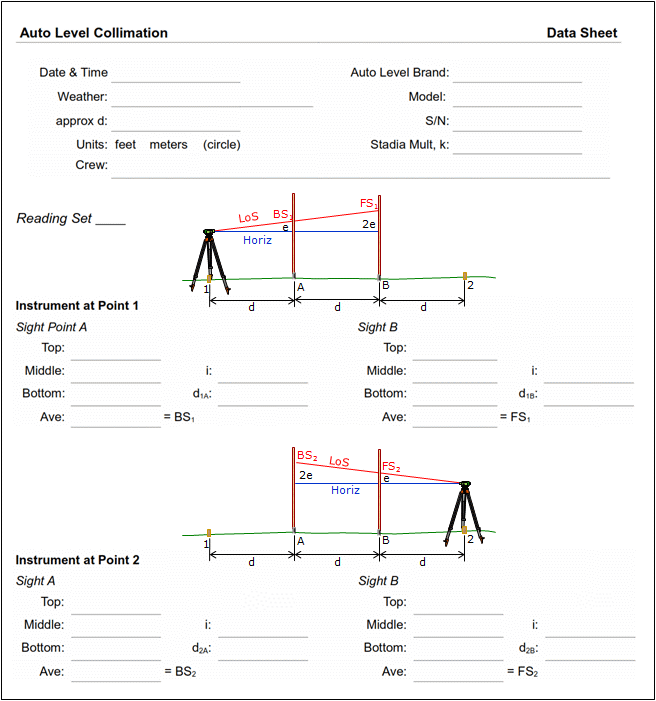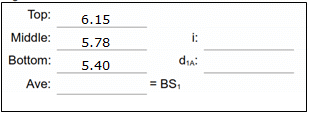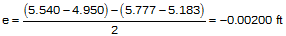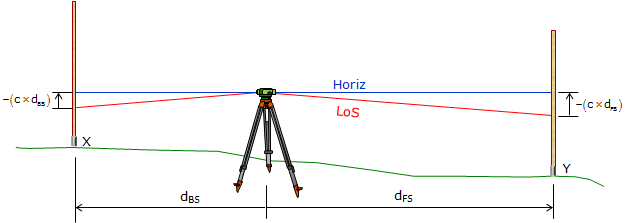1. Principle

A collimation error exists if the Line of Sight (LoS) is not horizotal when the instrument is leveled, Figure G-1. The LoS will be inclined or depressed and is caused by incorrect vertical location of the crosshairs.Figure G-1 Collimation Error

Level Collimation is the process of determining the error present in an instrument. Once the error is determined, the instrument can be adjusted or the error can be mathematically applied to each reading made with the instrument. The drawback to mathematical error resolution is that the sight length of each reading is necessary since the error is a function of distance.

2. Theory

Because collimation error cancels if BS and FS distances are balanced, the Level Collimation process purposely uses unbalanced sight distances. The elevation difference between two points is determined using a short BS / long FS condition followed by a long BS / short FS condition. If there is a collimation error, the two elevation differences will not be the same.

Because collimation error is a function of distance, the sight length for each reading is needed. The error is then stated as ratio (e.g. ft/ft) or angle above (+) or below (-) horizontal.

A baseline which consists of four evenly spaced points is established. The points should be on an approximately straight line and placed at a uniform interval of 75 to 100 ft, Figure G-2. Points 1 and 2 are instrument locations and are marked with wooden stakes; Points A and B are elevation points and are marked with stable pins.Figure G-2 Base Line

The instrument is set up at point 1 (it does not have to be exactly over the point) and readings are taken to points A and B.Figure G-3 First Setup

The instrument is moved to point 2 (again, it does not have to be exactly over the point) and readings are taken to points A and B.Figure G-4 Second Setup

The elevation difference point A to point B is:

from Point 1: BS– FS1

from Point 2: BS– FS2

Because of the error, e:

from Point 1: (BS– e) – (FS– 2e)

from Point 2: (BS– 2e) – (FS– e)

If we set the two differences equal and solve for e we get Equation G-1:Equation G-1

e is the total vertical error in a horizontal sight distance of d. Collimation error can be expressed as a ratio, Equation G-2, or angle, Equation G-3.

 ratioEquation G-2 angleEquation G-3

3. Three wire readings

Rather than set the four points accurately at an interval of d, it is easier to set them at the approximate interval and then measure the distances as the readings are made. This is done using the instrument's stadia crosshairs. An level has two additional horizontal crosshairs spaced equally above and below the main horizontal crosshair, Figure G-5.Figure G-5 Stadia Crosshairs

The instrument's manual will specify the multiplier (or stadia multiplier), k, for the instrument (it's also generally labeled in the case). In most cases the multiplier is 100 (to 3 sig fig).

The stadia interval, i, is the difference between the top and bottom stadia hair rod readings. Multiplying the rod interval by the instrument's multiplier gives the horizontal distance from the instrument to the rod:Equation G-4 d: horizontal distance k: stadia multiplier i: stadia intervalFigure G-6 Horizontal Distance from Stadia Crosshairs

Besides allowing us to determine the distance to a point, reading all three horizontal crosshairs provides a check on the rod reading: since they are equally spaced, the center hair reading should be the average of all three. The average may not be exactly the same as the center reading because all three readings are subject to random errors.

Example

The following shows a backsight on a point. The instrument used has a multiplier of 100.top: 4.31 middle: 4.16 bottom: 4.02 Figure G-7 Readig Stadia Crosshairs

Average: (4.31+4.16+4.02) / 3 = 4.163'   checks middle hair reading

The BS reading is 4.163' - note that we're carrying an additional decimal place since it is the average of three readings (also correct for sig fig).

i = 4.31-4.02 = 0.29'

The distance to the rod is 100(0.29') = 29'

When three-wire leveling the rod must be held vertical using a bubble. Waving the rod does not work, Figure G-8, since the lowest reading on the top and bottom stadia hairs do not occur when the rod is vertical.Figure G-8 Waving the Rod Introduces Errors

In the collimation process the distances from point 1 to A and B and from point 2 to A and B are mesaured by stadia.The measurements are used to compute an overall average d used to obtain the collimation error.Figure G-9 Base Line Point SpacingEqn (G-5)

4. Sample data sheet

The following data sheet can be used to record readings and perform the collimation computations both as a ratio (c) and vertical angle (θ).5. Example collimation check

Instrument used has a stadia multiplier of 100; rod units are feet.

A baseline was laid out with a cloth tape using an interval of approximately 75 feet.

Instrument set up at point 1

Sight rod on A

 Read and record the three crosshairs.Reduced readings: Average should be close to the middle reading, if not, then one or more readings incorrect; re-read. Check: d1A should be approx 75 ft, same as interval.It's important to reduce the readings immediately after taking them. The computations help identify if a reading error has been made. If there is an error, the equipment is still in place so the measurements can be quickly redone.

Sight rod on B

 Read and record the three crosshairs.Reduced readings Average should be close to the middle reading, if not, then one or more readings incorrect; re-read. Check: d1B should be approx 150 ft, twice the interval.The instrument is moved to Point 2 and the process repeated.The readings and computations are:

Sight rod on ASight rod on BCompute the error and distance from Equations G-1 and G-5:A negative error indicates theLoS is below horizontal.Using Equations (G-2) and (G-3) express collimation error as a ratio and angle:6. How much is acceptable?

Table G-1 shows the maximum allowable instrument collimation error for First, Second, and Third Order leveling.

 Table G-1From Standards and Specifications for Geodetic Control Networks, FGCS, 1984; Section 3.5 Geodetic Leveling.

The Standards and Specifications... also state that "Compensator-type instruments should be checked for proper operation at least every 2 weeks of use."

While these are control-level standards, they can serve as a guide for local applications. Local project control might use Third (or possibly Second) Order specifications while that for topographic control would be looser. Regardless, the error in an instrument is not known unless a collimation check is performed.

7. Collimation correction

Once the collimation error has been determined, there are three ways to deal with it

(1) Adjust the crosshairs

The reticule adjusting screws are located near the eyepiece under a cover. Removing the cover reveals the adjusting screws at the top and possibly bottom of the reticule (there are also side adjusting screws but they aren't necessary for this adjustment), Figure G-10Figure G-10 Reticule Adjusting Screws

If there is an upper and lower screw, then one must be first loosened and the other then tightened to move the crosshairs.

If there is only a single screw then only that screw is loosened or tightened.

How far to move the crosshairs?

For the previous example, we know that the total error in the BS from point 2 to A is: (152 ft)x(-0.0000266 ft/ft) = -0.004 ft.

The correct BS reading should be 5.540+0.004 = 5.544 ft

That means to remove the collimation error, we would use the adjusting screws to raise the crosshairs to a 5.544 ft BS reading.

Hmm... trying to see 0.004 ft at 152 ft... not too easy.

Probably best to leave the crosshairs alone and use one of the other two methods.

(2) Apply a correction to readings

Whenever the level is used, the correction could be applied to each reading made, Figure G-11.Figure G-11 Unbalance Sight Distances

The collimation error is compensated by multiplying the correction by the BS and FS length difference and adding it to the elevation difference.This requires the length of each sight be captured as the readings are taken. This is the case when three-wire leveling but generally not so when doing simple leveling using only the center hair unless paces are counted and recorded.

(3) Balance BS and FS distances

The simplest way to deal with the collimation error is to balance the BS and FS distances so the error cancels itself. No calculations are needed. Plus this method has the advantage of compensating other distance-based errors: refraction and curvature.